Request a Free Counselling Session from our Expert Mentor# NCERT Solution for Class Xth Maths Chapter 4 Quadratic Equations

Quadratic Equations is an important chapter in the Class Xth Maths syllabus, which lays the foundation for solving complex mathematical problems. This chapter deals with the concept of quadratic equations and their various applications in real-life situations. In this article, we will discuss the NCERT Solution for Class Xth Maths Chapter 4 Quadratic Equations by Swastik Classes. These solutions provide comprehensive explanations and step-by-step solutions to all the problems in the textbook, making it easier for students to understand the concepts and excel in their exams. In the following sections, we will provide an overview of the topics covered in this chapter and how the NCERT solutions by Swastik Classes can help students in their preparation.

## Answers of Maths NCERT solutions for Class Xth Maths Chapter 4 Quadratic Equations

Unit 4

Exercise 4.1

##### Q1 :

Check whether the following are quadratic equations: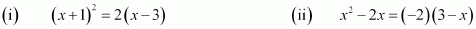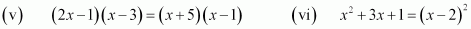Answer: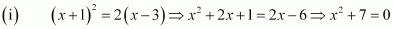It is of the form

Hence, the given equation is a quadratic equation.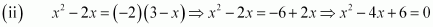It is of the form .

Hence, the given equation is a quadratic equation.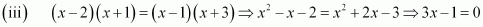It is not of the form .

Hence, the given equation is not a quadratic equation.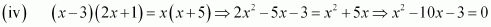It is of the form .

Hence, the given equation is not a quadratic equation.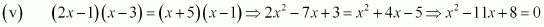It is of the form

Hence, the given equation is a quadratic equation

It is not of the form .

Hence, the given equation is not a quadratic equation.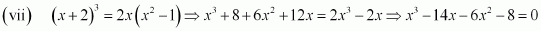It is not of the form

Hence, the given equation is not a quadratic equation.

It is of the form

Hence, the given equation is a quadratic equation.

##### Q2 :

Represent the following situations in the form of quadratic equations.

1. The area of a rectangular plot is 528 m2. The length of the plot (in metres) is one more than twice its breadth. We need to find the length and breadth of the plot.
2. The product of two consecutive positive integers is 306. We need to find the integers.
3. Rohan’s mother is 26 years older than him. The product of their ages (in years) 3 years from now will be

360. We would like to find Rohan’s present age.

1. A train travels a distance of 480 km at a uniform speed. If the speed had been 8 km/h less, then it would have taken 3 hours more to cover the same distance. We need to find the speed of the train.

1. Let the breadth of the plot be x m. Hence, the length of the plot is (2x + 1) m. Area of a rectangle = Length x Breadth

∴ 528 = x (2x + 1)

1. Let the consecutive integers be x and x + 1. It is given that their product is 306.
1. Let Rohan’s age be x. Hence, his mother’s age = x + 26 3 years hence,

Rohan’s age = x + 3

Mother’s age = x + 26 + 3 = x + 29

It is given that the product of their ages after 3 years is 360.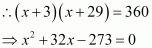1. Let the speed of the train be x km/h.

Time taken to travel 480 km =

In second condition, let the speed of train =   km/h

It is also given that the train will take 3 hours to cover the same distance.

Therefore, time taken to travel 480 km hrs Speed x Time = Distance

Exercise 4.2

##### Q1

Find the roots of the following quadratic equations by factorisation: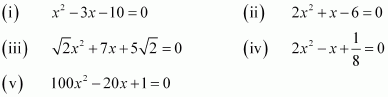Roots of this equation are the values for which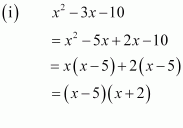∴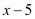= 0 or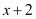=  i.e., x = 5 or x = – 2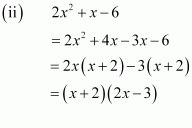Roots of this equation are the values for which

∴= 0 or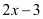= 0 i.e., x = – 2 or x =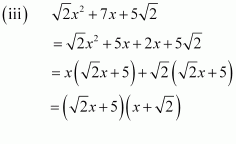Roots of this equation are the values for which

∴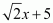= 0 or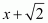= 0

i.e., x =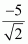or x =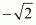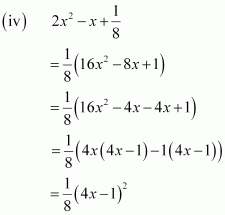Roots of this equation are the values for which

Therefore,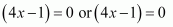i.e.,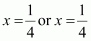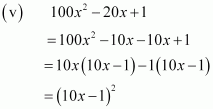Roots of this equation are the values for

Therefore,i.e.,

##### Q2

Solve the given situations mathematically.

1. John and Jivanti together have 45 marbles. Both of them lost 5 marbles each, and the product of the number of marbles they now have is 124. Find out how many marbles they had to start with.
2. A cottage industry produces a certain number of toys in a day. The cost of production of each toy (in rupees) was found to be 55 minus the number of toys produced in a day. On a particular day, the total cost of production was Rs 750. Find out the number of toys produced on that day.

(i) Let the number of John’s marbles be x. Therefore, number of Jivanti’s marble = 45 – x After losing 5 marbles,

Number of John’s marbles = x – 5

Number of Jivanti’s marbles = 45 – x – 5 = 40 – x

It is given that the product of their marbles is 124.

Either = 0 or x – 9 = 0 i.e., x = 36 or x = 9

If the number of John’s marbles = 36,

Then, number of Jivanti’s marbles = 45 – 36 = 9 If number of John’s marbles = 9, Then, number of Jivanti’s marbles = 45 – 9 = 36

(ii) Let the number of toys produced be x.

∴ Cost of production of each toy = Rs (55 – x)

It is given that, total production of the toys = Rs 750

Either

Hence, the number of toys will be either 25 or 30.

##### Q3

Find two numbers whose sum is 27 and product is 182.

Let the first number be x and the second number is 27 – x. Therefore, their product = x (27 – x)

It is given that the product of these numbers is 182.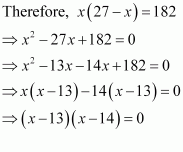Either  = 0 or x – 14 = 0 i.e., x = 13 or x = 14

If first number = 13, then Other number = 27 – 13 = 14 If first number = 14, then Other number = 27 – 14 = 13

Therefore, the numbers are 13 and 14.

##### *Q4

Find two consecutive positive integers, sum of whose squares is 365.

Let the consecutive positive integers be x and x + 1.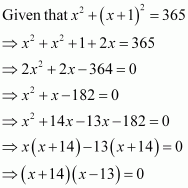Either Since the integers are positive, x can

only be 13.

∴ x + 1 = 13 + 1 = 14

Therefore, two consecutive positive integers will be 13 and 14.

##### *Q5

The altitude of a right triangle is 7 cm less than its base. If the hypotenuse is 13 cm, find the other two sides.

Let the base of the right triangle be x cm. Its altitude = (x – 7) cm

Either x – 12 = 0 or x + 5 = 0, i.e., x = 12 or x = – 5 Since sides are positive, x can only be 12.

Therefore, the base of the given triangle is 12 cm and the altitude of this triangle will be (12 – 7) cm = 5 cm.

*Q6

A cottage industry produces a certain number of pottery articles in a day. It was observed on a particular day that the cost of production of each article (in rupees) was 3 more than twice the number of articles produced on that day. If the total cost of production on that day was Rs 90, find the number of articles produced and the cost of each article.

Let the number of articles produced be x.

Therefore, cost of production of each article = Rs (2x + 3) It is given that the total production is Rs 90.

Either 2x + 15 = 0 or x – 6 = 0, i.e., x =    or x = 6

As the number of articles produced can only be a positive integer, therefore, x can only be 6. Hence, number of articles produced = 6

Cost of each article = 2 × 6 + 3 = Rs 15

Exercise 4.3

##### Q1 :

Find the roots of the following quadratic equations, if they exist, by the method of completing the square: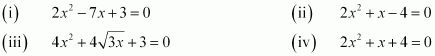Answer :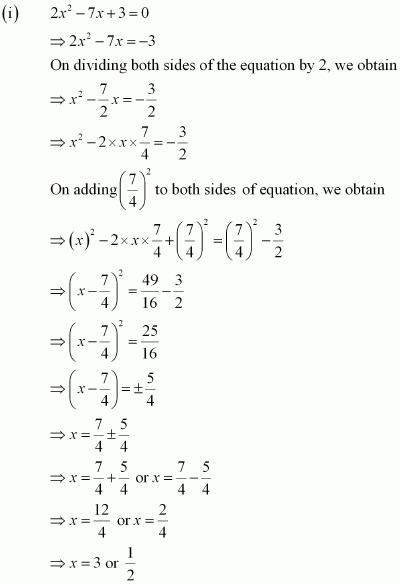(ii)

There are two solution:

(iii)

##### Q2

Find the roots of the quadratic equations given in Q.1 above by applying the quadratic formula.

(i)

Roots

or

or

(ii)

Roots

Hence there are two solutions

and

##### Q3

Find the roots of the following equations: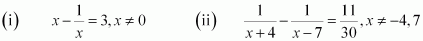Q4:

The sum of the reciprocals of Rehman’s ages, (in years) 3 years ago and 5 years from now is . Find his present age.

Let the present age of Rehman be x years.

Three years ago, his age was (x – 3) years.

Five years hence, his age will be (x + 5) years.

It is given that the sum of the reciprocals of Rehman’s ages 3 years ago and 5 years from now is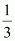.

However, age cannot be negative. Therefore, Rehman’s present age is 7 years.

Q5:

In a class test, the sum of Shefali’s marks in Mathematics and English is 30. Had she got 2 marks more in Mathematics and 3 marks less in English, the product of their marks would have been 210. Find her marks in the two subjects.

Let the marks in Maths be x.

Then, the marks in English will be 30 – x. According to the given question,

If the marks in Maths are 12, then marks in English will be 30 – 12 = 18

If the marks in Maths are 13, then marks in English will be 30 – 13 = 17

##### Q6:

The diagonal of a rectangular field is 60 metres more than the shorter side. If the longer side is 30 metres more than the shorter side, find the sides of the field.

Let the shorter side of the rectangle be x m.

Then, larger side of the rectangle = (x + 30) m

However, side cannot be negative. Therefore, the length of the shorter side will be 90 m.

Hence, length of the larger side will be (90 + 30) m = 120 m

##### *Q7:

The difference of squares of two numbers is 180. The square of the smaller number is 8 times the larger number. Find the two numbers.

Let the larger and smaller number be x and y respectively. According to the given question,

However, the larger number cannot be negative as 8 times of the larger number will be negative and hence, the square of the smaller number will be negative which is not possible.

Therefore, the larger number will be 18 only.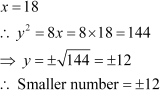Therefore, the numbers are 18 and 12 or 18 and – 12.

*Q8:

A train travels 360 km at a uniform speed. If the speed had been 5 km/h more, it would have taken 1 hour less for the same journey. Find the speed of the train.

Let the speed of the train be x km/hr.

Time taken to cover 360 km

According to the given question,

However, speed cannot be negative. Therefore, the speed of train is 40 km/hr.

##### Two water taps together can fill a tank in hours. The tap of larger diameter takes 10 hours less than the smaller one to fill the tank separately. Find the time in which each tap can separately fill the tank.

Let the time taken by the smaller pipe to fill the tank be x hr. Time taken by the larger pipe

Part of tank filled by smaller pipe in 1 hour

Part of tank filled by larger pipe in 1 hour

It is given that the tank can be filled in hours by both the pipes together. Therefore,

Time taken by the smaller pipe cannot be = 3.75 hours. As in this case, the time taken by the larger pipe will be negative, which is logically not possible.

Therefore, time taken individually by the smaller pipe and the larger pipe will be 25 and 25 – 10 =15 hours respectively.

##### *Q10:

An express train takes 1 hour less than a passenger train to travel 132 km between Mysore and Bangalore (without taking into consideration the time they stop at intermediate stations). If the average speeds of the express train is 11 km/h more than that of the passenger train, find the average speed of the two trains.

Let the average speed of passenger train be x km/h.

Average speed of express train = (x + 11) km/h

It is given that the time taken by the express train to cover 132 km is 1 hour less than the passenger train to cover the same distance.

Speed cannot be negative.

Therefore, the speed of the passenger train will be 33 km/h and thus, the speed of the express train will be 33 + 11 = 44 km/h.

##### *Q-11:

Sum of the areas of two squares is 468 m2. If the difference of their perimeters is 24 m, find the sides of the two squares.

Let the sides of the two squares be x m and y m.

Therefore, their perimeter will be 4x and 4y respectively and their areas will be x2 and y2 respectively.

It is given that 4x – 4y = 24

x – y = 6

x = y + 6

However, side of a square cannot be negative.

Hence, the sides of the squares are 12 m and (12 + 6) m = 18 m

Exercise – 4.4

##### Q1

Find the nature of the roots of the following quadratic equations. If the real roots        exist, find them;

(I)

(II)

(III)

We know that for a quadratic equation discriminant is b2 – 4ac.

If two distinct real roots

If two equal real roots

If no real roots

(I)

Comparing this equation with ,  we obtain

Discriminant

= – 31

As b2 – 4ac < 0,

Therefore, no real root is possible for the given equation.

(II)

Comparing this equation with we obtain

Discriminant

= 48 – 48 = 0

As b2 – 4ac = 0,

Therefore, real roots exist for the given equation and they are equal to each other. And the roots will be

Therefore, the roots are

(III)

Comparing this equation with we obtain

a = 2, b = – 6, c = 3

Discriminant

= 36 – 24 = 12

As b2 – 4ac > 0,

Therefore, distinct real roots exist for this equation as follows.

Therefore, the roots are

Or

##### *Q2:

Find the values of k for each of the following quadratic equations, so that they have two equal roots.

(I)

(II) kx(x – 2) + 6 = 0

We know that if an equation ax2 + bx + c = 0 has two equal roots, its discriminant (b2 – 4ac) will be 0.

(I) 2x+kx + 3 = 0

Comparing equation with ax2 + bx + c = 0, we obtain

a = 2, b = k, c = 3

Discriminant

= k2 – 24

For equal roots, Discriminant = 0

(II) or

Comparing this equation with we obtain

Discriminant

For equal roots,

Either or

or

However, if k = 0, then the equation will not have the terms and ‘x‘. Therefore, if this equation has two equal roots, k should be 6 only.

##### *Q3 :

Is it possible to design a rectangular mango grove whose length is twice its breadth, and the area is 800 m2? If so, find its length and breadth.

Let the breadth of mango grove be l.

Length of mango grove will be 2l.

Area of mango grove = (2l) (l)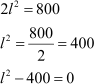Comparing this equation with we obtain

Discriminant Here,

Therefore, the equation will have real roots. And hence, the desired rectangular mango grove can be designed.

However, length cannot be negative.

Therefore, breadth of mango grove = 20 m

Length of mango grove = 2 × 20 = 40 m

*Q4:

Is the following situation possible? If so, determine their present ages. The sum of the ages of two friends is 20 years. Four years ago, the product of their ages in years was 48.

Let the age of one friend be x years.

Age of the other friend will be (20 – x) years.

4 years ago, age of 1st friend = (x – 4) years

And, age of 2nd friend = (20 – x – 4)= (16 – x) years

Given that,

(x – 4) (16 – x) = 48

16x – 64 – x2 + 4x = 48

-x2 + 20x – 112 = 0

x2 – 20x + 112 = 0

Comparing this equation with ax2 + bx + c = 0, we obtain

a = 1, b = -20, c = 112

Discriminant

As

Therefore, no real root is possible for this equation and hence, this situation is not possible.

*Q5:

Is it possible to design a rectangular park of perimeter 80 and area 400 m2? If so find its length and breadth.

Let the length and breadth of the park be l and b.

Perimeter = 2 (l + b) = 80

l + b = 40 or, b = 40 – l

Area

Comparing this equation with

we obtain

Discriminate

As

Therefore, this equation has equal real roots.

And hence, this situation is possible. Root of this equation,

Therefore, length of park, l = 20 m

And breadth of park, b = 40 – l = 40 – 20 = 20 m

NCERT Solutions for Class 10 Maths Chapter 4- Quadratic Equations
A 1 mark question was asked from Chapter 4 Quadratic Equations in the year 2018. However, in the year 2017, a total of 13 marks were asked from the topic Quadratic Equations. Therefore, students need to have a thorough understanding of the topic. The topics and sub-topics provided in this chapter include:

List of Exercises we covered in NCERT Solutions for Class 10 Maths Chapter 4:
Exercise 4.1 Solutions– 2 Questions
Exercise 4.2 Solutions– 6 Questions
Exercise 4.3 Solutions– 11 Questions
Exercise 4.4 Solutions– 5 Questions In a quadratic equation, x represents an unknown form and a, b, c are the known values. An equation to be quadratic “a” should not be equal to 0. The equation is of the form ax2 + bx + c = 0. The values of a, b, and c are always real numbers. A quadratic equation can be calculated by completing the square. A quadratic equation has:

Two different real roots.
No real roots.
Frequently Asked Questions on NCERT Solutions for Class 10 Maths Chapter 4
How many exercises are there in NCERT Solutions for Class 10 Maths Chapter 4?
The fourth Chapter of NCERT Solutions for Class 10 Maths has 4 exercises. The first exercise deals with the topic of determining the quadratic equations, second exercise has questions of finding the roots of quadratic equations by factorization, third exercise deals with finding the roots of quadratic equations by completing squares and last exercise has the question based on the nature of the roots. By solving these exercises students are able to answer all the questions based on quadratic equations.
Is SWC’S website providing answers for NCERT Solutions for Class 10 Maths Chapter 4 Quadratic Equations?
Yes, you can avail the PDFs of NCERT Solutions for Class 10 Maths Quadratic Equations. These solutions are formulated by expert faculty at SWC’S in an unique way. Also they provide solutions for Class 1 to 12 NCERT Textbooks in free PDFs. Who wish to score high in exams are advised to solve the NCERT Textbook.
Mention the important concepts that you learn in NCERT Solutions for Class 10 Maths Chapter 4 Quadratic Equations?
The concepts presented in NCERT Solutions for Class 10 Maths Chapter 4 Quadratic Equations are meaning and definition of quadratic equations, finding the roots of quadratic equations by factorization, finding the roots of quadratic equations by completing squares and nature of the roots.